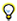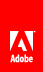# Drawing lines and curves

Flash Player 9 and later, Adobe AIR 1.0 and later

All drawing that you do with a Graphics instance is based on basic drawing with lines and curves. Consequently, all ActionScript drawing must be performed using the same series of steps:

• Define line and fill styles

• Set the initial drawing position

• Draw lines, curves, and shapes (optionally moving the drawing point)

• If necessary, finish creating a fill

## Defining line and fill styles

To draw with the graphics property of a Shape, Sprite, or MovieClip instance, you must first define the style (line size and color, fill color) to use when drawing. Just like when you use the drawing tools in Adobe® Flash® Professional or another drawing application, when you’re using ActionScript to draw you can draw with or without a stroke, and with or without a fill color. You specify the appearance of the stroke using the lineStyle() or lineGradientStyle() method. To create a solid line, use the lineStyle() method. When calling this method, the most common values you’ll specify are the first three parameters: line thickness, color, and alpha. For example, this line of code tells the Shape named myShape to draw lines that are 2 pixels thick, red (0x990000), and 75% opaque:

`myShape.graphics.lineStyle(2, 0x990000, .75);`

The default value for the alpha parameter is 1.0 (100%), so you can leave that parameter off if you want a completely opaque line. The lineStyle() method also accepts two additional parameters for pixel hinting and scale mode; for more information about using those parameters see the description of the Graphics.lineStyle() method in the ActionScript 3.0 Reference for the Adobe Flash Platform .

To create a gradient line, use the lineGradientStyle() method. This method is described in Creating gradient lines and fills .

If you want to create a filled shape, you call the beginFill() , beginGradientFill() , beginBitmapFill() , or beginShaderFill() methods before starting the drawing. The most basic of these, the beginFill() method, accepts two parameters: the fill color, and (optionally) an alpha value for the fill color. For example, if you want to draw a shape with a solid green fill, you would use the following code (assuming you’re drawing on an object named myShape ):

`myShape.graphics.beginFill(0x00FF00);`

Calling any fill method implicitly ends any previous fill before starting a new one. Calling any method that specifies a stroke style replaces the previous stroke, but does not alter a previously specified fill, and vice versa.

Once you have specified the line style and fill properties, the next step is to indicate the starting point for your drawing. The Graphics instance has a drawing point, like the tip of a pen on a piece of paper. Wherever the drawing point is located, that is where the next drawing action will begin. Initially a Graphics object begins with its drawing point at the point 0, 0 in the coordinate space of the object on which it’s drawing. To start the drawing at a different point, you can first call the moveTo() method before calling one of the drawing methods. This is analogous to lifting the pen tip off of the paper and moving it to a new position.

With the drawing point in place you draw using a series of calls to the drawing methods lineTo() (for drawing straight lines) and curveTo() (for drawing curved lines).While you are drawing, you can call the moveTo() method at any time to move the drawing point to a new position without drawing.

While drawing, if you have specified a fill color, you can close off the fill by calling the endFill() method. If you have not drawn a closed shape (in other words, if at the time you call endFill() the drawing point is not at the starting point of the shape), when you call the endFill() method the Flash runtime automatically closes the shape by drawing a straight line from the current drawing point to the location specified in the most recent moveTo() call. If you have started a fill and not called endFill() , calling beginFill() (or one of the other fill methods) closes the current fill and starts the new one.

## Drawing straight lines

When you call the lineTo() method, the Graphics object draws a straight line from the current drawing point to the coordinates you specify as the two parameters in the method call, drawing with the line style you have specified. For example, this line of code puts the drawing point at the point 100, 100 then draws a line to the point 200, 200:

```myShape.graphics.moveTo(100, 100);
myShape.graphics.lineTo(200, 200);```

The following example draws red and green triangles with a height of 100 pixels:

```var triangleHeight:uint = 100;
var triangle:Shape = new Shape();

// red triangle, starting at point 0, 0
triangle.graphics.beginFill(0xFF0000);
triangle.graphics.moveTo(triangleHeight / 2, 0);
triangle.graphics.lineTo(triangleHeight, triangleHeight);
triangle.graphics.lineTo(0, triangleHeight);
triangle.graphics.lineTo(triangleHeight / 2, 0);

// green triangle, starting at point 200, 0
triangle.graphics.beginFill(0x00FF00);
triangle.graphics.moveTo(200 + triangleHeight / 2, 0);
triangle.graphics.lineTo(200 + triangleHeight, triangleHeight);
triangle.graphics.lineTo(200, triangleHeight);
triangle.graphics.lineTo(200 + triangleHeight / 2, 0);

## Drawing curves

The curveTo() method draws a quadratic Bézier curve. This draws an arc that connects two points (called anchor points) while bending toward a third point (called the control point). The Graphics object uses the current drawing position as the first anchor point. When you call the curveTo() method, you pass four parameters: the x and y coordinates of the control point, followed by the x and y coordinates of the second anchor point. For example, the following code draws a curve starting at point 100, 100 and ending at point 200, 200. Because the control point is at point 175, 125, this creates a curve that moves to the right and then downward:

```myShape.graphics.moveTo(100, 100);
myShape.graphics.curveTo(175, 125, 200, 200);```

The following example draws red and green circular objects with a width and height of 100 pixels. Note that due to the nature of the quadratic Bézier equation, these are not perfect circles:

```var size:uint = 100;
var roundObject:Shape = new Shape();

// red circular shape
roundObject.graphics.beginFill(0xFF0000);
roundObject.graphics.moveTo(size / 2, 0);
roundObject.graphics.curveTo(size, 0, size, size / 2);
roundObject.graphics.curveTo(size, size, size / 2, size);
roundObject.graphics.curveTo(0, size, 0, size / 2);
roundObject.graphics.curveTo(0, 0, size / 2, 0);

// green circular shape
roundObject.graphics.beginFill(0x00FF00);
roundObject.graphics.moveTo(200 + size / 2, 0);
roundObject.graphics.curveTo(200 + size, 0, 200 + size, size / 2);
roundObject.graphics.curveTo(200 + size, size, 200 + size / 2, size);
roundObject.graphics.curveTo(200, size, 200, size / 2);
roundObject.graphics.curveTo(200, 0, 200 + size / 2, 0);